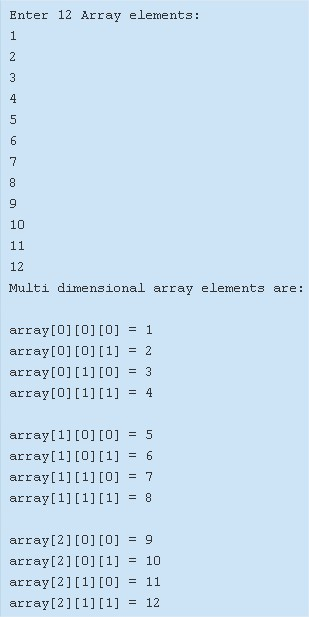# Multi Dimensional Array In Java – Tutorial & Program

All the methods will be explained with sample programs and suitable examples. The compiler has also been added so that you understand the whole thing clearly.

• Using Standard Method
• Using For Loop
• Using Scanner Class
• Using String

An array, as we all know, is a collection of multiple elements of the same data type. Arrays are normally used to store information of one particular type of variable.

As the name of the title suggests, multi-dimensional means any particular entity having 3 or more than 3 dimensions in reality.

Similarly, a multi-dimensional array in Java usually has 3 or more defined indexes, but in reality, one particular row of elements have another multitude of elements defined on their names.

Basically, you can have a 3×3 or a bigger matrix of elements to define a multi-dimensional array.As you can see as per the image uploaded above, firstly, you need to specify the number of rows and columns respectively.

The example roughly explains the structure of a 3×4 matrix.

So, the total number of elements that will be entered is 12.

The elements that are entered are as follows:

1 2 3 4 5 6 7 8 9 10 11 12

These elements will be arranged accordingly and the multi-dimensional array will be printed for you.

Thus, the numerous methods that are used to carry out the same task in Java are:

## Multi Dimensional Array – Using Standard Method

Output:

### Multidimensional – Using For Loop

1. To insert the elements in to multi dimensional array a[][][], we are using for loop.

for i=0 to 2

for j=0 to 1

for k=0 to 1

insert the element at a[i][j][k].

2) Print the elements using for loops

for i=0 to 2

for j=0 to 1

for k=0 to 1

print a[i][j][k]

Output:

#### Using Scanner Class

1.  To insert  the elements in to multi dimensional array array[][][]  we are using scanner class.
2. for i=0 to 2
3. for j=0 to 1
4. for k=0 to 1
5. sc.nextInt() method reads the entered number and inserted the number in to array[i][j][k] .

Output:

##### Using String
1. Initialize the strings as elements in to the multi dimensional string array str[][][].

2) Print the strings from multidimensional array str[][][].

for i=0 to 2

for j=0 to 1

for k=0 to 1

print the string available at the index str[i][j][k].

Output:

x

## Plus Star Pattern Java Program | Patterns

Java program to print plus star pattern program – We have written the below print/draw ...